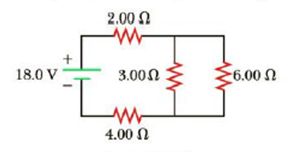Chapter 18, Problem 16P

Chapter
Section
Textbook Problem

(a) Is it possible to reduce the circuit shown in Figure P18.16 to a single equivalent resistor connected across the battery? Explain. (b) Find the current in the 2.00-Ω resistor. (c) Calculate the power delivered by the battery to the circuit.Figure P18.16

(a)

To determine
The possibility of the circuit shown in figure P18.6 is reduced into a single equivalent resistor.

Explanation

Given Info: The two parallel resistor network are R3=3.00Ω and R4=6.00Ω .

Explanation:

The following figure shows the resistor network can be reduced to a single equivalent resistance in the below steps:

Formula to calculate the equivalent resistance of the parallel combination is,

1Rp=1R3+1R4

• Rp is the equivalent resistance of the parallel combination,
• R3 and R4 is the resistors,

Rewrite the above relation.

Rp=R3R4R3+R4

Substitute 3.00Ω for R3 and 6.00Ω for R4 .

Rp=(3.00Ω)(6.00Ω)3

(b)

To determine
The current passing through the 2.00Ω resistor.

(c)

To determine
The power delivered by the battery to the given circuit.

Still sussing out bartleby?

Check out a sample textbook solution.

See a sample solution

The Solution to Your Study Problems

Bartleby provides explanations to thousands of textbook problems written by our experts, many with advanced degrees!

Get Started

Find more solutions based on key concepts